Monday - Friday 8:30am to 7:00pm EST 800-510-8325

Cushion Fabric Yardage Guide

How to Determine Fabric Requirements

Length
Length is the sum of the longest side's measurement; the product of your thickness multiplied by 2; and the product of multiplying the number of breaks by thickness, then by 2. If ties, include an additional 2″.
Depth
If the sum of the depth's (short side) measurement and the thickness is greater than 25″, double the fabric allot for your "length″ measurement above.
If the sum of the depth's measurement and the thickness is greater than 52″, then multiply the width by 2.6.

Fabric Repeats

“X” equals the horizontal measurement of a fabric repeat. “Y” is the vertical measurement of a fabric repeat.

• When the sum of X and Y is greater than 2″ and less than or equal to 14″, multiply its adjusted depth by 1.1.
• When the sum of X and Y is greater than 14″ and less than or equal to 19″, multiply its adjusted depth by 1.15.
• When the sum of X and Y is greater than 19″ and less than or equal to 27″, multiply its adjusted depth by 1.2.
• When the sum of X and Y is greater than 27″ and less than or equal to 36″, multiply its adjusted depth by 1.25.
• When the sum of X and Y is greater than 36″ and less than or equal to 45″, multiply its adjusted depth by 1.3.
• When the sum of X and Y is greater than 45″, multiply its adjusted depth by 1.35.

Treat cording separate regardless if it is the same as the main fabric and order a minimum of 1 yard.

Directional Fabrics

We run all directional fabrics vertical (front to back) on a product unless otherwise stated. Please note that vertical run fabrics may cause seams on cushions longer than 50″.

Fabric Yardage Measurement Examples

Seat Cushion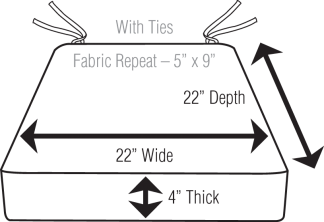Length
22″ (longest side) + 8″ (4″ thickness x 2) + 2″ (ties) = 32″ Length

Depth
22″ (short side) + 4″ (thickness) = 26″ Depth
Since 26″ is greater than 25″, double its length to 64″ (adjusted length).

Fabric Repeat
5″ + 9″ equals 14″, thus you will multiply your adjusted length by 1.1 for a total of 70.40″. Divide 70.40″ by 36″ for a total of 1.96 yards. Round this number to 2 yards.

Cording
Provide 1 yard.

Total Amount of Fabric
Send a total of 3 yards.

Bench Cushion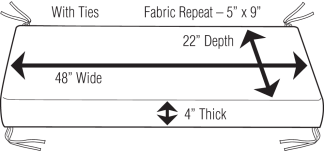Length
48″ (longest side) + 8″ (4″ thickness x 2) + 2″ (ties) = 58″ Length

Depth
22″ (short side) + 4″ (thickness) = 26″ Depth
Since 26″ is greater than 25″, double its length to 116″ (adjusted length).

Fabric Repeat
5″ + 9″ equals 14″, thus you will multiply your adusted length by 1.1 for a total of 127.6″. Divide 127.6″ by 36″ for a total of 3.54 yards. Round this number to 4 yards.

Cording
Provide 1 yard.

Total Amount of Fabric
Send a total of 5 yards.

Chaise Cushion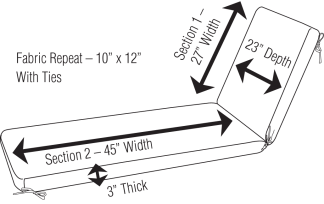Length
72″ (27″ + 45″, longest side) + 6″ (3″ thickness x 2) + 6″ (1 break x 3″ thickness x 2) + 2″ (ties) = 86″ Length

Depth
23″ (short side) + 3″ (thickness) = 26″ Depth
Since 26″ is greater than 25″, double its length to 172″ (adjusted length).

Fabric Repeat
10″ + 12″ equals 22″, thus you will multiply your adusted length by 1.2 for a total of 206.4″. Divide 206.4″ by 36″ for a total of 5.73 yards. Round this number to 6 yards.

Cording
Provide 1 yard.

Total Amount of Fabric
Send a total of 7 yards.

Double Chaise Cushion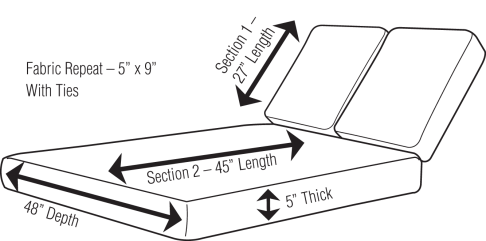Length
72″ (27″ + 45″, longest side) + 10″ (5″ thickness x 2) + 10″ (1 break x 5″ thickness x 2) + 2″ (ties) = 94″ Length

Depth
48″ (short side) + 5″ (thickness) = 53″ Depth
Since 53″ is greater than 52″, multiply its length by 2.6 to equal 244.4″ (adjusted length).

Fabric Repeat
5″ + 9″ equals 14″, thus you will multiply your adusted width by 1.1 for a total of 268.8″. Divide 268.8″ by 36″ for a total of 7.47 yards. Round this number to 8 yards.

Cording
Provide 1 yard.

Total Amount of Fabric
Send a total of 9 yards.

Deep Seating Sofa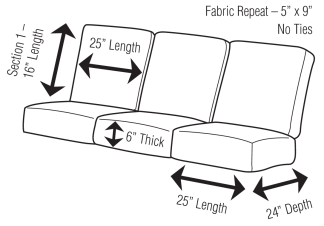Length
25″ (longest side) + 12″ (6″ thickness x 2) = 37″ Length

Depth
24″ (short side) + 6″ (thickness) = 30″ Depth
Since 30″ is greater than 25″, multiply its length by 2 to equal 74″ (adjusted length).

Fabric Repeat
5″ + 9″ equals 14″, thus you will multiply your adusted length of 74″ by 1.1 for a total of 81.4″. Divide 81.4″ by 36″ for a total of 2.26 yards.

Multiply 2.26 yards by 3 cushions, equaling 6.78 yards.

Now multiply your adusted length of 37″ by 1.1 for a total of 40.7″. Divide 40.7″ by 36″ for a total of 1.13 yards. Multiply 1.13 yards by 3 cushions, equals 3.39 yards. 6.78 + 3.39 = 10.17 yards. Round this number to 11 yards.

Cording
Provide 1 yard.

Total Amount of Fabric
Send a total of 12 yards.

Company Information